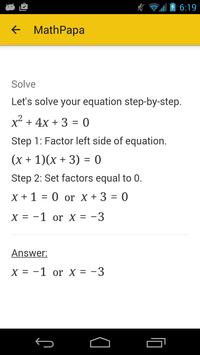##MathPapa - Algebra Calculator   Version:  1.1.6

### Application Description:

SOLVE your algebra problems step-by-step with MathPapa!

FEATURES:
- Solves linear equations and quadratic equations.
- Solves linear and quadratic inequalities.
- Graphs equations.
- Order of operations step-by-step.
- Evaluates expressions.
- Solves systems of two equations.

WORS OFFLINE!

STEP-BY-STEP SOLUTIONS:

Get help on your algebra problems with the Math Papa Algebra Calculator!

HOW TO USE THE CALCULATOR:

Just type your problem into the text box.

For example, enter 3x+5=17 into the text box to get a step-by-step explanation of how to solve 3x+5=17.

MATH SYMBOLS:

Here are some symbols that the Math Papa calculator understands:

- (Subtraction)
- (Multiplication)
/ (Division)
^ (Exponent: "raised to the power")
(Square Root)
|x| (Absolute Value of x)

## Conclusion

MathPapa - Algebra Calculator was filed under the Education category and was reviewed in softlookup.com and receive 5/5 Score.
MathPapa - Algebra Calculator has been tested by our team against viruses, spyware, adware, trojan, backdoors and was found to be 100% clean. We will recheck MathPapa - Algebra Calculator when updated to assure that it remains clean.

### MathPapa - Algebra Calculator user Review

Please review MathPapa - Algebra Calculator application and submit your comments below. We will collect all comments in an effort to determine whether the MathPapa - Algebra Calculator software is reliable, perform as expected and deliver the promised features and functionalities.